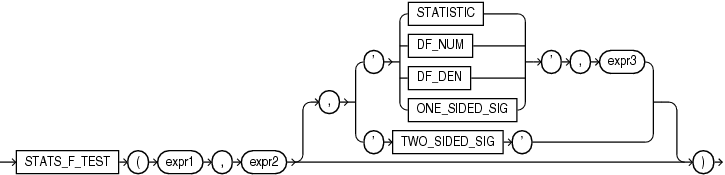## STATS_F_TEST

SyntaxDescription of the illustration stats_f_test.eps

Purpose

`STATS_F_TEST` tests whether two variances are significantly different. The observed value of f is the ratio of one variance to the other, so values very different from 1 usually indicate significant differences.

This function takes two required arguments: `expr1` is the grouping or independent variable and `expr2` is the sample of values. The optional third argument lets you specify the meaning of the `NUMBER` value returned by this function, as shown in Table 7-5. For this argument, you can specify a text literal, or a bind variable or expression that evaluates to a constant character value. If you omit the third argument, then the default is `'TWO_SIDED_SIG'`.

Appendix C in Oracle Database Globalization Support Guide for the collation determination rules for `STATS_F_TEST`

Table 7-5 STATS_F_TEST Return Values

Argument Return Value Meaning

`'STATISTIC'`

The observed value of f

`'DF_NUM'`

Degree of freedom for the numerator

`'DF_DEN'`

Degree of freedom for the denominator

`'ONE_SIDED_SIG'`

One-tailed significance of f

`'TWO_SIDED_SIG'`

Two-tailed significance of f

The one-tailed significance is always in relation to the upper tail. The final argument, `expr3`, indicates which of the two groups specified by `expr1` is the high value or numerator (the value whose rejection region is the upper tail).

The observed value of f is the ratio of the variance of one group to the variance of the second group. The significance of the observed value of f is the probability that the variances are different just by chance—a number between 0 and 1. A small value for the significance indicates that the variances are significantly different. The degree of freedom for each of the variances is the number of observations in the sample minus 1.

STATS_F_TEST Example

The following example determines whether the variance in credit limit between men and women is significantly different. The results, a p_value not close to zero, and an f_statistic close to 1, indicate that the difference between credit limits for men and women are not significant.

```SELECT VARIANCE(DECODE(cust_gender, 'M', cust_credit_limit, null)) var_men,
VARIANCE(DECODE(cust_gender, 'F', cust_credit_limit, null)) var_women,
STATS_F_TEST(cust_gender, cust_credit_limit, 'STATISTIC', 'F') f_statistic,
STATS_F_TEST(cust_gender, cust_credit_limit) two_sided_p_value
FROM sh.customers;

VAR_MEN  VAR_WOMEN F_STATISTIC TWO_SIDED_P_VALUE
---------- ---------- ----------- -----------------
12879896.7   13046865  1.01296348        .311928071```